# How to edit a Tank's speed/horsepower and firerate?

As the title suggest i am trying to figure out how to edit the speed/horsepower and firerate of a tank for some funny/silly experiments i want to try out but i am unable to figure out how to change them. I am trying it on a BT7 tank and i can’t find any option to edit any of what i mentioned above.

1 Like

Extra question: What does Vehicle_hp and Vehicle_max_hp do?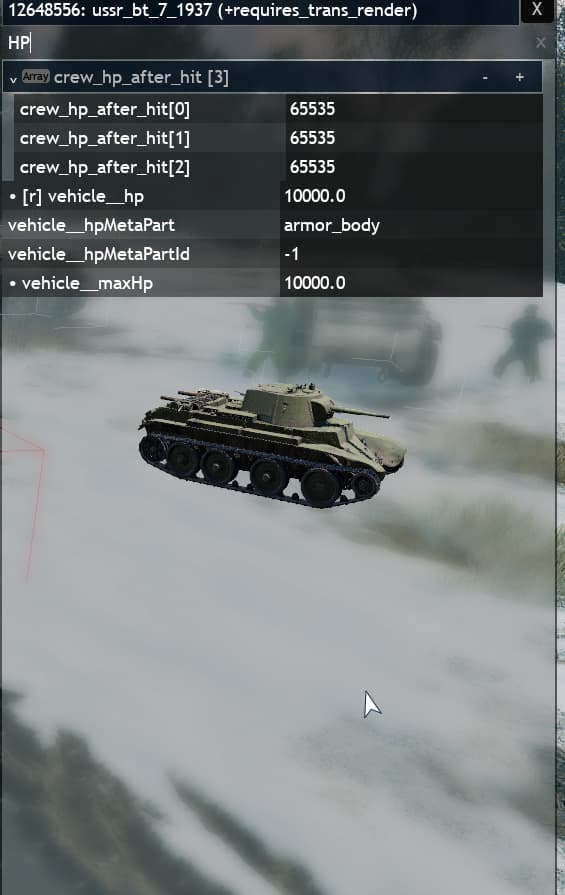1 Like

You can a bit edit vehicle stats chainging vehicle_mods__… properties: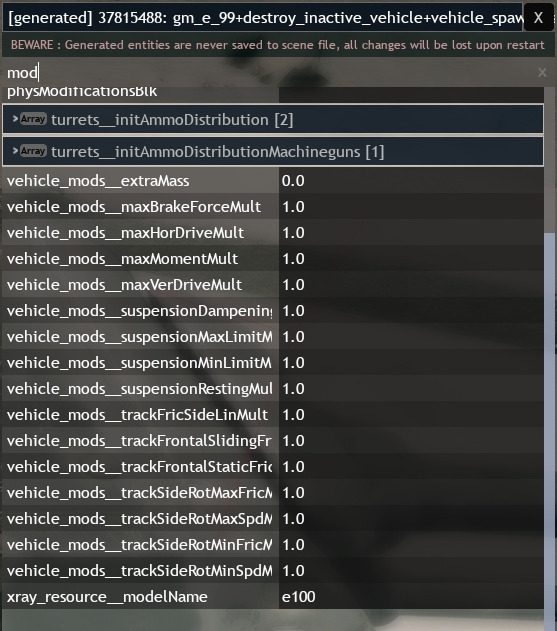But as I know… They contains some limits.

Best way, but at same time hard is… Edit vehicle phys/engine. Change vehicle_net_phys__blk property.

Yeah… It should be as seperate blk file. For create it you need to find your mod folder in userGameMods. After that create text file and change file type from .txt to .blk. After that open it and write code. All what I can to tell… That this file contains this structure like it:

``````VehiclePhys{
skipUpdateOnSleep:b=yes

Mass{
Empty:r=1320.0
Fuel:r=43.0
TakeOff:r=1320.0
momentOfInertia:p3=0.324786, 1.168099, 1.012251
CenterOfGravity:p3=0.0, 0.5, 0.0
CenterOfGravityClampY:p2=0.0, 0.5
trackMass:r=4.0
engineInertialMass:r=5.0
}

tracks{
animationMultiplier:r=0.5
height:r=0.0
width:r=0.125
trackPhysMat:t="wheel"
}

collisionProps{
cls_body_01:t="convex_hull"
cls_body_02:t="convex_hull"
cls_body_03:t="convex_hull"
cls_body_04:t="convex_hull"
collisionMaterial:t="walkRay"
}

engine{
horsePowers:r=54.0
maxRPM:r=2800.0
minRPM:r=900.0
rpmTau:r=0.1
minTorqueLimiter:r=0.0
}

mechanics{
maxBrakeForce:r=7900.0
mainGearRatio:r=4.444
sideGearRatio:r=1.0
tracked:b=no
gearType:t="differential"

gearRatios{
ratio:r=-7.82
ratio:r=0.0
ratio:r=6.4
ratio:r=3.09
ratio:r=1.69
ratio:r=1.0
}
}

suspension{
suspensionOffsets:p3=-0.15, -0.15, 0.12
sinkableDist:r=0.07
defaultDampeningForce:p2=4000.0, 4000.0
hasSuspensionOnDriveGear:b=yes
dampeningRelaxationRatio:r=0.8
dampeningCompressionRatio:r=0.8
recalcWtms:b=yes

steeringDeflection{
wheel_l_01:r=45.0
wheel_r_01:r=45.0
steeringTau:r=10.0
steeringSpd:r=1.2
steeringNeutralSpd:r=1.8
steeringNegativeMult:r=3.0
steeringSpdMult:p4=10.0, 50.0, 1.0, 0.3
}

toeDeflection{
wheel_l_01:r=-0.5
wheel_r_01:r=0.5
}

wheelsWidth{
wheel_l_01:r=0.125
wheel_l_drive:r=0.125
wheel_r_01:r=0.125
wheel_r_drive:r=0.125
}

driven{
wheel_l_01:b=yes
wheel_r_01:b=yes
wheel_l_drive:b=yes
wheel_r_drive:b=yes
}

handBraken{
wheel_l_drive:b=yes
wheel_r_drive:b=yes
}

wheelsDamageWeight{
wheel_l_01:r=0.25
wheel_r_01:r=0.25
wheel_l_drive:r=0.25
wheel_r_drive:r=0.25
}
}
}

DamageParts{
formatVersion:i=2
armorClass:t="RHA_tank"
hp:r=100.0

body{

body{
createSecondaryShatters:b=no
armorThickness:r=0.1
armorThrough:r=0.15
}
}

engine{
hp:r=55.0

engine{
hitTestType:t="inner"
malfunctionDamage:r=0.7
fireProtectionHp:r=5.0
externalArmor:b=no
minRelHpSelfRepair:r=0.5
maxRelHpSelfRepair:r=0.5
selfRepairDelay:r=0.0
selfRepairTime:r=-1.0
splashPenetrationToArmorThreshold:r=0.33
pressureDamageMult:r=0.0
napalmDamageMult:r=0.0
sonicDamageMult:r=0.0
vital:b=yes
movement:b=yes
fire:b=yes
armorClass:t="armor_tank_engine"
hp:r=90.0
armorThickness:r=50.0
restrainDamage:r=0.5
}
}

transmission{
hp:r=10.0

transmission{
createSecondaryShatters:b=no
armorThickness:r=0.3
armorThrough:r=10.0
}
}

wheels{
hp:r=55.0

wheel_r_01{
hitTestType:t="exclude"
malfunctionDamage:r=0.7
fireProtectionHp:r=-1.0
externalArmor:b=no
minRelHpSelfRepair:r=0.5
maxRelHpSelfRepair:r=0.5
selfRepairDelay:r=0.0
selfRepairTime:r=-1.0
splashPenetrationToArmorThreshold:r=0.33
pressureDamageMult:r=0.0
napalmDamageMult:r=0.0
sonicDamageMult:r=0.0
vital:b=yes
armorClass:t="car_wheels"
hp:r=55.0
armorThickness:r=10.0
restrainDamage:r=0.5
}

wheel_l_01{
hitTestType:t="exclude"
malfunctionDamage:r=0.7
fireProtectionHp:r=-1.0
externalArmor:b=no
minRelHpSelfRepair:r=0.5
maxRelHpSelfRepair:r=0.5
selfRepairDelay:r=0.0
selfRepairTime:r=-1.0
splashPenetrationToArmorThreshold:r=0.33
pressureDamageMult:r=0.0
napalmDamageMult:r=0.0
sonicDamageMult:r=0.0
vital:b=yes
armorClass:t="car_wheels"
hp:r=55.0
armorThickness:r=10.0
restrainDamage:r=0.5
}

wheel_l_drive{
hitTestType:t="exclude"
malfunctionDamage:r=0.7
fireProtectionHp:r=-1.0
externalArmor:b=no
minRelHpSelfRepair:r=0.5
maxRelHpSelfRepair:r=0.5
selfRepairDelay:r=0.0
selfRepairTime:r=-1.0
splashPenetrationToArmorThreshold:r=0.33
pressureDamageMult:r=0.0
napalmDamageMult:r=0.0
sonicDamageMult:r=0.0
vital:b=yes
armorClass:t="car_wheels"
hp:r=55.0
armorThickness:r=10.0
restrainDamage:r=0.5
}

wheel_r_drive{
hitTestType:t="exclude"
malfunctionDamage:r=0.7
fireProtectionHp:r=-1.0
externalArmor:b=no
minRelHpSelfRepair:r=0.5
maxRelHpSelfRepair:r=0.5
selfRepairDelay:r=0.0
selfRepairTime:r=-1.0
splashPenetrationToArmorThreshold:r=0.33
pressureDamageMult:r=0.0
napalmDamageMult:r=0.0
sonicDamageMult:r=0.0
vital:b=yes
armorClass:t="car_wheels"
hp:r=55.0
armorThickness:r=10.0
restrainDamage:r=0.5
}
}
}

DamageEffects{

part{
name:t="engine"
name:t="transmission"

onKill{
fire:r=1.0
fHitCritical:b=yes
}
}

part{
name:t="body"

onHit{
damageType:t="cumulative"
expl:r=1.0
damage:r=100.0
fHitCritical:b=yes
}

onHit{
damageType:t="explosion"
expl:r=1.0
damage:r=100.0
fHitCritical:b=yes
}
}
}

MetaParts{

armor_body{
hp:r=400.0
part:t="engine"
part:t="transmission"
part:t="body"

effect{
killEffect:t="expl"
kill:b=yes
}
}
}
``````

(GAZ_67 code).

After that in game you need to edit vehicle_net_phys__blk property. Add link to your new file. %ugm is your mod folder. So link maybe will be looks like: %ugm/your_vehicle. Keep in mind that file must be only with lowercase words!

If you want edit stats for all vehicles not for only one/placed on map… Then you also need to create entities.blk file. And edit vehicle/tank game object code. Entities.blk code structure and example.

I don’t know, but it doesn’t much affect to vehicle/tanks lifetime. Because it can be destroyed if somebody destroy all modules. You can edit hp in damage_model__blk property (link to file is same that you use in vehicle_net_phys__blk, but without :VehiclePhys!

1 Like

There two ways. One of ways - edit tank turret entity in entities.blk.
Another one (if you want to increase firerate only for certain vehicles that placed on your map) is edit turretsInitialComponents property.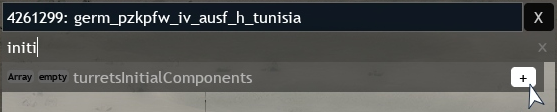You will need to create object, because tank contains some turrets, not only one. First object is first turret (it’s usually tank cannon) and e.t.c.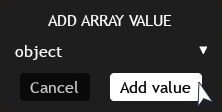After that you will need to click + on new line. And write property that you want to edit.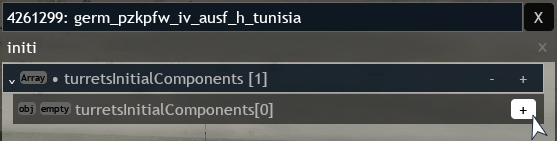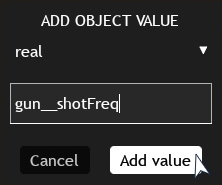Using Find Entity you can find what turret entities tank uses.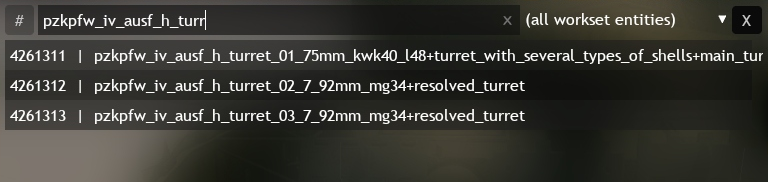(You can select them and check properties in panel).

Or you can do it by checking turret_control__turretInfo property.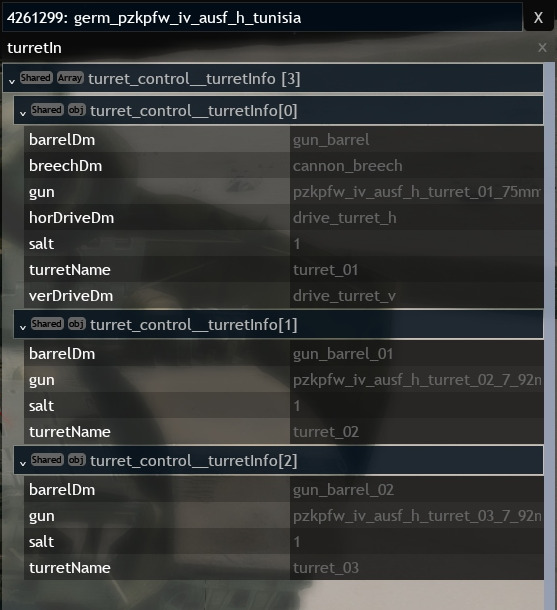If you want to edit damage/projectile stats then you will need to edit gun__shells property.

Yeah. It almost same how in vehicle_net_phys__blk/damage_model__blk properties.

There some code/structure example, but yeah… It’s more about infantry weapons. Anyways…

``````mass:r=0.008
cartridgeMass:r=0.0228
caliber:r=0.0074
speed:r=730.0
maxDistance:r=2000.0
bulletType:t="ball"
hitPowerMult:r=13.8
effectiveDistance:r=350.0
relativeVelHitShift:p2=300.0, 1000.0
relativeVelArmorShift:p2=200.0, 1000.0

hitpower{
HitPower10m:p2=1.0, 10.0
HitPower100m:p2=0.82, 100.0
HitPower200m:p2=0.67, 200.0
HitPower300m:p2=0.55, 300.0
HitPower400m:p2=0.45, 400.0
HitPower500m:p2=0.37, 500.0
HitPower1000m:p2=0.12, 1000.0
HitPower1500m:p2=0.06, 1500.0
}

armorpower{
ArmorPower0m:p2=1.0, 10.0
ArmorPower1500m:p2=0.1, 1500.0
}

visual{
range_percent_of_ammo:p2=100.0, 0.0
traceFreq:i=1
tracer:t="7mmYellow"
trail:t="smallTrail"
}

tracer{
ignitionTime:r=0.002
smokeColor:c=255, 230, 179, 204
smokeTime:r=0.01
luminosity:r=10.0
burnTime:r=1.8
}
``````

(Rifle).

``````spawn{
minCount:i=18
maxCount:i=18
}
``````

Will multiple bullets amount. Like shotgun shell.

3 Likes

Thanks!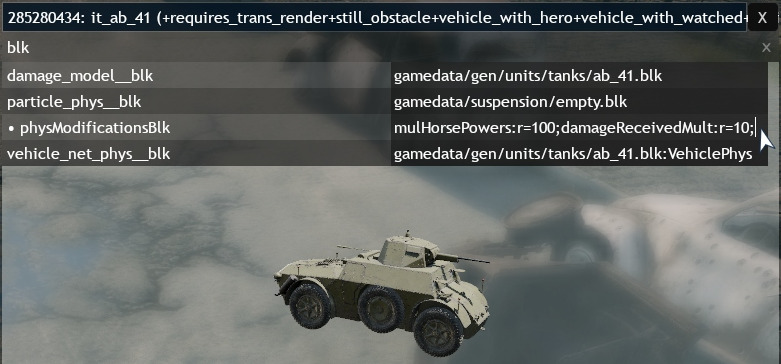``````mulCdminFusel:r=0.833333;mulCompressorMaxP:r=0.93;cutProbabilityMult:r=1;damageReceivedMult:r=1;mulMass:r=0.947867;mulCdminTail:r=0.934579;radiatorEffMul:r=0.95;mulOswEffNumber:r=0.9;mulHorsePowers:r=0.9;mulCdmin:r=0.875657;